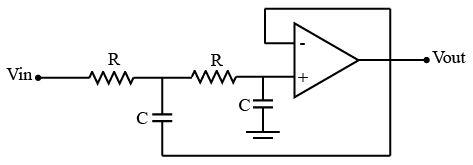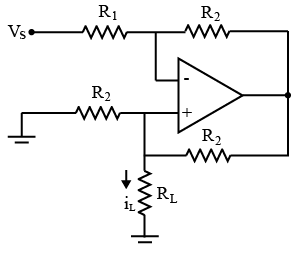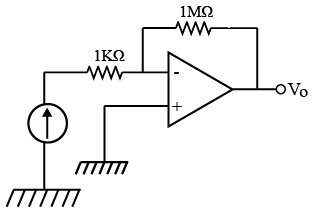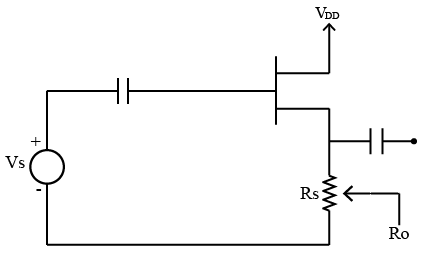# ISRO Scientist or Engineer Electronics 2008

Instructions

For the following questions answer them individually

Question 1

# The circuit shown in figure is aQuestion 2

# In the op-amp circuit given in figure, the load current $$i_L$$ isQuestion 3

# An op-amp has an offset voltage of 1 mV and is ideal in all other respects. If this op-amp is used in the circuit shown in figure, the output voltage will beQuestion 4

# For the circuit shown above if $$g_m = 3 \times 10^{-3}$$ and $$R_s = 3000 Ω$$, thenn the approximate value of $$R_o$$ isQuestion 5

# Where does the operating point of a class-B power amplifier lie?Question 6

# The Laplace transform of $$e^{-2t}$$ isQuestion 7

# The system with characteristic equation $$s^4 + 3s^3 + 6s^2 + 9s + 12 = 0$$Question 8

# Compared to field effect photo transistors, bipolar photo transistors areQuestion 9

# The output VI characteristics of an enhancement type MOSFET hasQuestion 10

# Which of the following relation is valid?Where MTBF = Mean Time Between Failures MITTF = Mean Time To Failures MTTR = Mean Time To RepairOR